Open in App
Not now

# Equilibrium of Bodies

• Last Updated : 20 Sep, 2022

The laws of motion, which are the foundation of old-style mechanics, are three explanations that portray the connections between the forces following up on a body and its movement. They were first expressed by English physicist and mathematician Isaac Newton. The motion of an item is related to the forces operating on it by Newton’s equations of motion. According to the first law, until a force acts on an item, it will not alter its motion. According to the second law, an object’s force equals its mass times its acceleration.

First Law Of Motion

According to Newton’s first law, Everybody continues to be in its state of rest or uniform motion until and unless an external force acts on the body. This law is also called as Law of Inertia. This is the most practical law which we can experience daily while driving a bike or travelling on a bus.

Second Law Of Motion

According to Newton’s second law, For an object of constant mass, the rate of change of momentum is the force acting on the body. Thus the product of mass and acceleration gives the magnitude of the force acting on the body.

F = d/dt(mv)

= m.(dV/dt)

= m.a

Hence, F = m.a m/s2

Third Law Of Motion

According to Newton’s third law, Every action on a body has an equal and opposite reaction. Example: Shooting using a rifle, Frictional force acting on the shoe while we walk.

## Equilibrium Of Bodies

Equilibrium in material science is the framework’s condition when neither its condition of movement nor its inside energy changes with time. Consider an object of mass ‘M’ and it is said to be in equilibrium when the sum of all the external forces acting on the mass ‘M’ is equal to zero. However, for an unbending body to be in equilibrium, the body ought to have both rotational and translational equilibrium which means the sum of net forces, net moments and external torque acting on the body should be zero.

### Static Equilibrium

Static equilibrium is the body’s actual state where all external torque and force on the body are zero, and the body is in the condition of rest. Static equilibrium is studied on objects which are at rest. The free body diagram of the mass ‘M’ should give net forces acting on the body equal to zero.

Examples:

• Chair resting on the floor.
• Fruits basket resting on the table.
• Stool resting on the floor.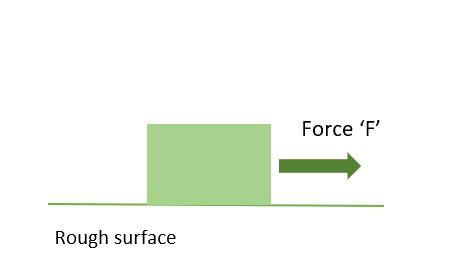Force acting on the body at rest.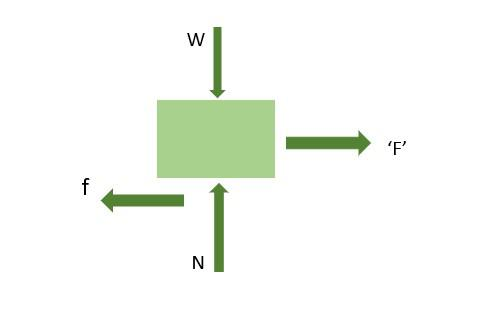FBD of the body in Static Equilibrium

For Static Equilibrium,

Net forces acting in the Y direction are equal to zero.

W(weight)=N(Normal Reaction)

Net forces acting in the X direction are equal to zero.

f(Frictional Force)=F(Applied force)

Considering the state and stability of the body, static equilibrium is further classified into three groups, namely:

1. Stable Equilibrium
2. Unstable Equilibrium
3. Neutral Equilibrium

Let’s study all of these in detail.

### Stable Equilibrium

A stable equilibrium is a kind of static equilibrium which exits when a point force acts on a body after that the body can attain its original position.

Example: Considering a cone of radius and height ‘H’, now place the cone on a smooth horizontal surface with its base touching the horizontal surface and then tilt it. It can be observed that the cone will retain its original position. This is a standard example of stable equilibrium.

### Unstable Equilibrium

Unstable equilibrium is a kind of static equilibrium which exits when a point force acts on a body after that the body can’t attain its original position.

Example: Considering a cone of radius and height ‘H’, now invert the cone and place it on a smooth horizontal surface and tilt it. It can be observed that the cone will not retain its original position. This is a standard example of unstable equilibrium.

### Neutral Equilibrium

If a ball is pushed marginally to roll, it will neither return to its unique position nor push ahead. It will stop after some time, in this kind of situation neutral equilibrium exists.

Example: Considering a cone of radius and height ‘H’, now place the cone with its slant face along the horizontal surface and then disturb it. It can be observed that the cone comes to rest after a certain time but it can’t attain its original position. This is a standard example of neutral equilibrium.

### Dynamic Equilibrium

Dynamic equilibrium is the body’s actual state where all external torque and force on the body are zero, and the body is in uniform motion. The free body diagram of the mass ‘M’ should give net forces acting on the body equal to zero.

Examples:

1. The motion of the toy globe about its axis.
2. The motion of a ball on a smooth floor.
3. The motion of planets around the sun,

## Solved Examples on Equilibrium of Bodies

Example 1. Calculate the force acting on a body of mass 2 kg with an acceleration of 3 m/s2.

Solution:

We have,

m = 2

a = 3

Using the formula we have,

F = ma

= 2 (3)

= 6 N

Example 2. Three forces P, Q, and R are in equilibrium with each other acting at a place. Angles between P and Q, Q and R, and P and R have a ratio of 1:2:3. Find the ratio of the forces, P:Q:R.

Solution:

Suppose the angles are a, 2a and 3a.

=> x + 2x + 3x = 360o

=> x = 60o

Using Lamis theorem we get,

P/sin 120o = Q/sin 180o = R/sin 60o

P/(√3/2) = Q/0 = R/(√3/2)

So, we get P:Q:R = √3/2 : 0 : √3/2.

Example 3. A body is subjected to two perpendicular forces of 4 N and 3 N. Find the magnitude of the resultant force on the body.

Solution:

We have,

F1 = 4

F2 = 3

Using the formula we have,

F = √(F12 + F22)

= √(42 + 32)

= √25

= 5 N

Example 4. Calculate the mass of a body whose acceleration is 2 m/s2 subjected to two perpendicular forces of 8 N and 6 N.

Solution:

We have,

a = 2

F1 = 8

F2 = 6

Using the formula we have,

F = √(F12 + F22)

= √(82 + 62)

= √100

= 10 N

We know,

m = F/a

= 10/2

= 5 kg

Example 5. Calculate the acceleration of a body whose mass is 3 kg subjected to two perpendicular forces of 5 N and 12 N.

Solution:

We have,

m = 3

F1 = 5

F2 = 12

Using the formula we have,

F = √(F12 + F22)

= √(52 + 122)

= √169

= 13 N

We know,

a = F/m

= 13/3

= 4.33 m/s2

## FAQs on Equilibrium of Bodies

Question 1: Represent stable equilibrium pictorially.

Considering a cone of radius and height ‘H’, now place the cone on a smooth horizontal surface with its base touching the horizontal surface and then tilt it. It can be observed that the cone will retain its original position. This is a standard example of stable equilibrium.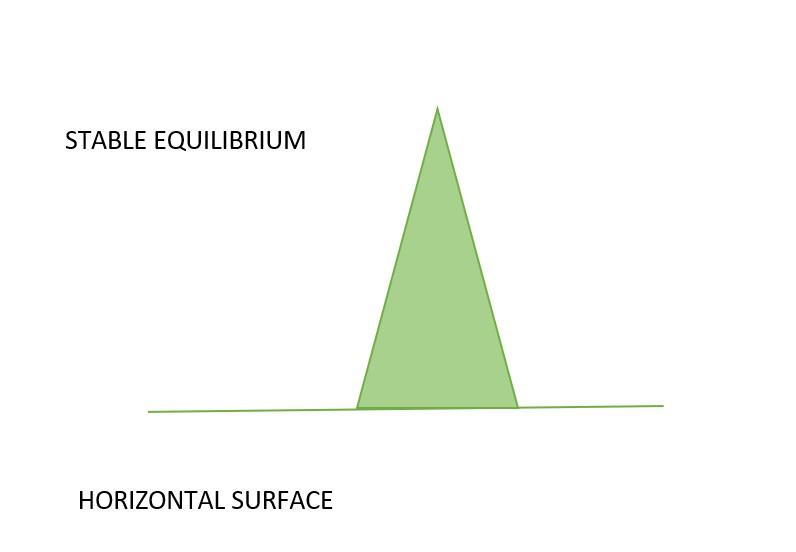Stable Equilibrium

Question 2: Represent Unstable equilibrium pictorially.

Considering a cone of radius and height ‘H’, now invert the cone and place it on a smooth horizontal surface and tilt it. It can be observed that the cone will not retain its original position. This is a standard example of unstable equilibrium.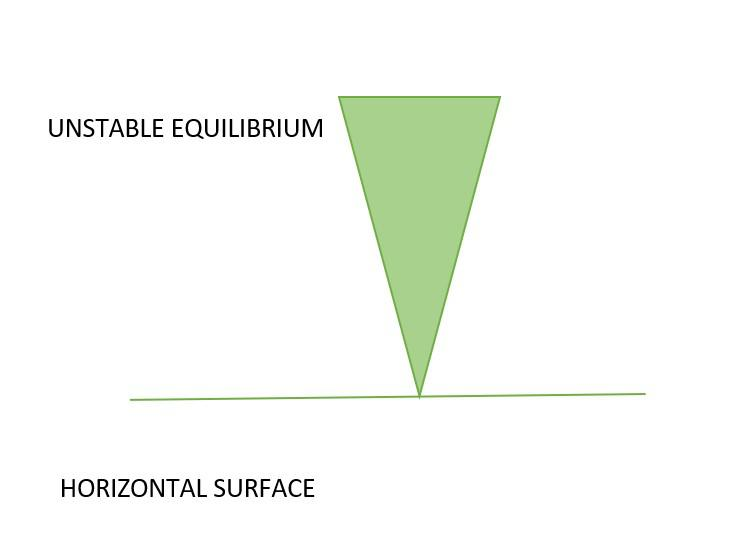Unstable Equilibrium

Question 3: Represent Neutral equilibrium pictorially.

Considering a cone of radius and height ‘H’, now place the cone with its slant face along the horizontal surface and then disturb it. It can be observed that the cone comes to rest after a certain time but it can’t attain its original position. This is a standard example of neutral equilibrium.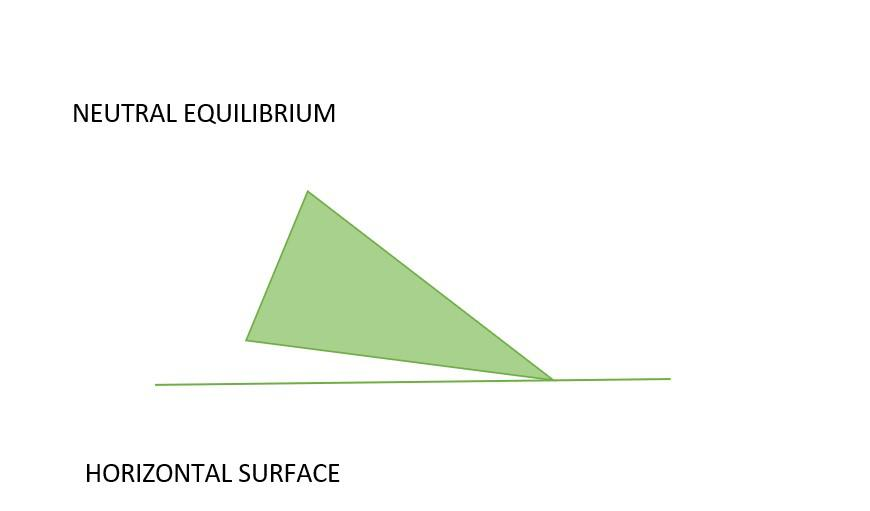Neutral Equilibrium

Question 4: What is the connection between potential energy and conditions of equilibrium?